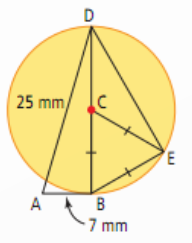The Learning Point‎ > ‎Mathematics‎ > ‎

## 1.Triangles and Congruence

Theorems:
1.1 Definition:Two triangles are congruent if their corresponding sides are equal in length and their corresponding angles are equal in size.

1.2 There are five basic condition that can be used to compare the congruency of triangles.They are as following:

1.SAS(Side-Angle-Side):If two pairs of sides of two triangles are equal in length, and the included angles are equal in measurement, then the triangles are congruent.It is illustrated by the folowing figure.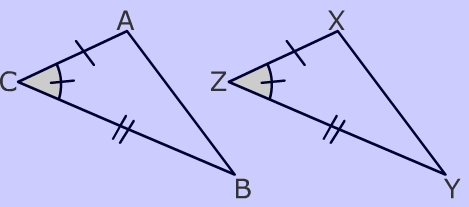2.SSS(Side-Side-Side):If three pairs of sides of two triangles are equal in length, then the triangles are congruent.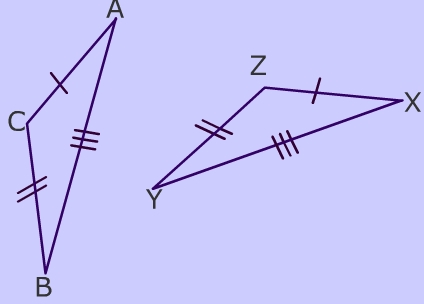3.ASA(Angle-Side-Angle):If two pairs of angles of two triangles are equal in measurement, and the included sides are equal in length, then the triangles are congruent.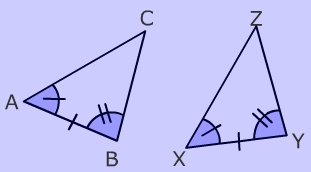4.AAS(Angle-Angle-Side):If two pairs of angles of two triangles are equal in measurement, and a pair of corresponding non-included sides are equal in length, then the triangles are congruent.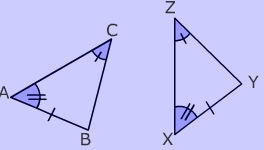5.RHS(Right-angle-Hypotenuse-Side):If two right-angled triangles have their hypotenuses equal in length, and a pair of shorter sides are equal in length, then the triangles are congruen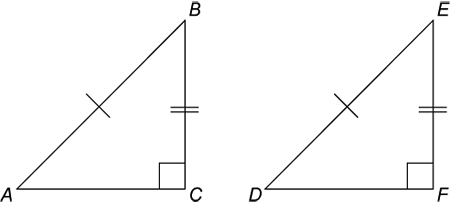1.3 Problems:
Q1.In the figure below, PX and QY are perpendicular to PQ and PX = QY. Show that AX = AY.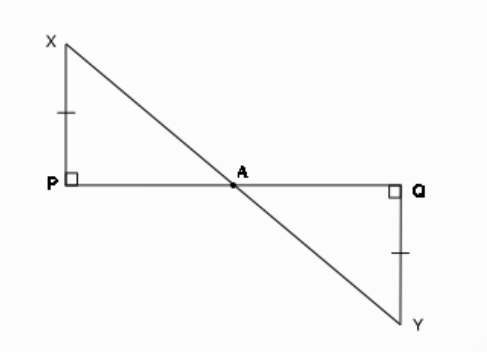Q2.In figure below, ∆ABC is a right triangle in which ∠B = 90° and D is the midpoint of AC.Prove that BD = ½ AC.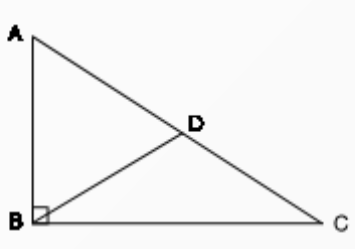Q3.In following figure,  ∠B =  ∠C and AB = AC. Prove that  ∆ABE  ≅ ∆ACD. Hence show that CD = BE.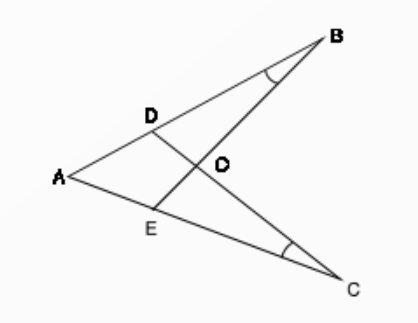Q4.ABC is an isosceles triangle in which AB = AC. If BD ⊥ AC and CE ⊥ AB, prove that BD = CE.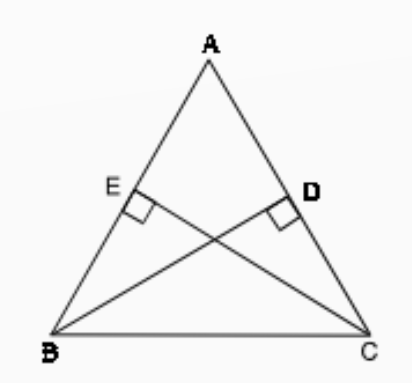Q5.In the following Figure, ∆ABC and ∆CDE are such that BC = CE and AB = DE. If ∠B = 60°, ∠ACE = 30° and ∠D = 90°, then prove that the two triangles are congruent.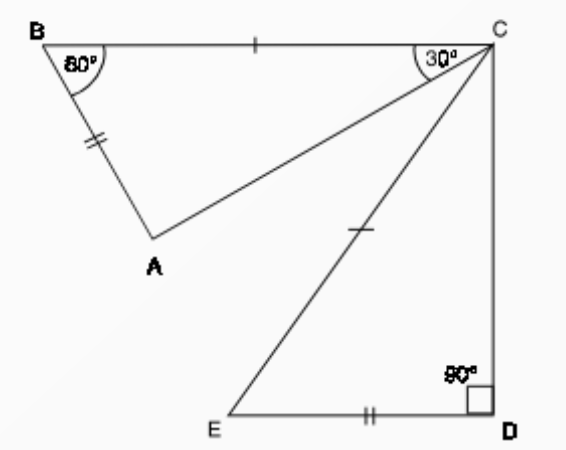Q6.In the following Fig., AB is the longest side and DC is the shortest
side of a quadrilateral ABCD. Prove that  ∠C > ∠A and ∠D > ∠B.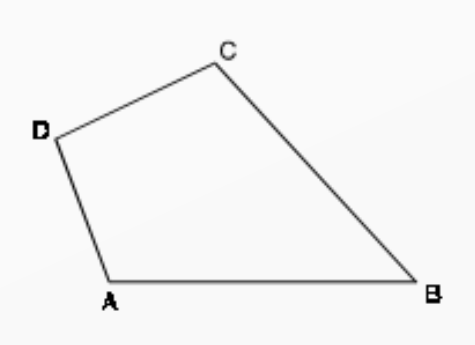## 2.Similarity of Triangles

Theorems:
2.1Two figures are similar if one is the image of the other under a transformation from the plane into itself that multiplies all distances by the same positive scale factor.

2.2Definition:Two triangles are similar if the following this are hold:
a)Their corresponding angles are equal.
b)Their corresponding sides are equal.

We say that  ∆ABC is similar to ∆DEF and denote it by writing
∆ABC ~ ∆DEF.

The symbol ‘~’ stands for the phrase “ is similar to”
If ∆ABC ~ ∆DEF, then by definition
∠A = ∠D, ∠B = ∠E, ∠D = ∠F and AB/DE = BC/EF = CA/FD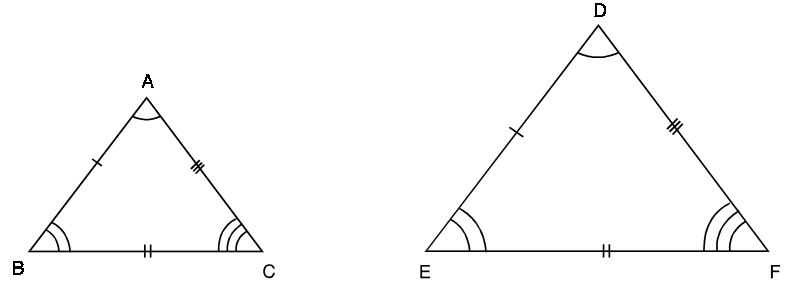2.3 It can be said that all congruent triangles are similar, but all similar triangles are not congruent. The only difference is that triangles are proportional by a scalar constant k, whereas in congruence that scalar is of unit value.

2.4 Criteria for similarity:
1.AAA(Angle-Angle-Angle):If in two triangles, the corresponding angles are equal then the triangles are similar.In can be easily seen in the figure below.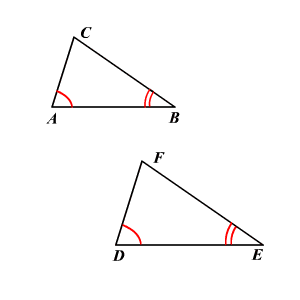2.SSS(Side-Side-Side):If the corresponding sides of two triangles are proportional then the triangles are similar. It is shown that the ratio of the corresponding sides are in the ratio of ½.

3. SAS(Side-Angle-Side):If one angle of a triangle is equal to one angle of the other triangle and the sides containing these angles are proportional, then the triangles are similar.

2.7 Basic Proportionality theorem:
a)If a line is drawn parallel to one side of a triangle, the other two sides of the triangle are divided proportionally.
b)If a line divides any two sides of a triangle in the same ratio, the line is parallel to third side of the triangle.

2.6 Area of similar Triangle theorem: In two similar triangles, the ratio of their areas is the square of the ratio of their sides. In the figure shown below all the blue triangles are are similar to the larger triangle and it can be seen the above theorem holds.

2.7 Problems:

Q1.In figure below, ∆ABC ~ ∆PQR. If AC = 4.8 cm, AB = 4 cm and PQ = 9 cm, find PR.

Q2.In Figure below, ABC is a right triangle with  ∠A = 90° and  ∠C = 30°. Show that ∆DAB ~ ∆DCA ~ ∆ACB.

Q3.In two similar triangles ABC and PQR, if the corresponding altitudes AD and PS are in the ratio of 4 : 9, find the ratio of the areas of ∆ABC and  ∆PQR.

Q4.In Figure below, ABC is a triangle in which DE || BC. If AB = 6 cm and AD = 2 cm, find the ratio of the area of ∆ADE and trapezium DBCE.

Q5.If the ratio of the areas of two similar triangles is 16 : 25, find the ratio of their corresponding sides.

Q6.The sides AB and AC of a triangle are 6 cm and 8 cm. The bisector AD of ∠A intersects the opposite side BC in D such that BD = 4.5 cm. Find the length of segment CD.

## 3. Midpoint theorem

Theorem:
3.1 Midpoint theorem:In any triangle, a segment joining the midpoints of any two sides will be parallel to the third side and half its length.In the figure below D and E are the midpoints of the sides shown.Thus from theorem DE || AB and DE= ½ AB.

3.2 Converse of midpoint theorem: If a segment bisects one side of a triangle and is parallel to another side, then it bisects the third side as well.

3.3 Equal Intercept theorem:  If two intersecting lines are cut by parallel lines, the line segments cut by the parallel lines from one of the lines are proportional to the corresponding line segments cut by them from the other line.In the following figure DE || BC, thus from the theorem

3.4 Problems:

Q1.In the figure below, E is the mid point of a median AD in triangle ABC and BE is produced to meet AC at F. Show that AF=1/3AC.

Q2.In PQR, A, B and C are the mid points of QR, RP and PQ respectively. If the lengths of sides PQ, QR and RP are 7cm, 8cm and 9 cm respectively, find the perimeter of ABC.

Q3.In ΔABC in the figure before, AD is the median and DE || AB. Prove that BE is another median.

Q4.ABCD is a parallelogram in which P, Q, R and S are the mid points of the sides AB, BC, CD and DA respectively. AC is a diagonal, show that PQRS is a parallelogram.

Q5.In the fihure shown the segments has the values as shown, and FA || CN, find the value of CN, AO and CO.

## 4.Pythagoras Theorem:

4.1 Theorem:In any right triangle, the area of the square whose side is the hypotenuse (the side opposite the right angle) is equal to the sum of the areas of the squares whose sides are the two legs (the two sides that meet at a right angle). It is illustrated in the figure below: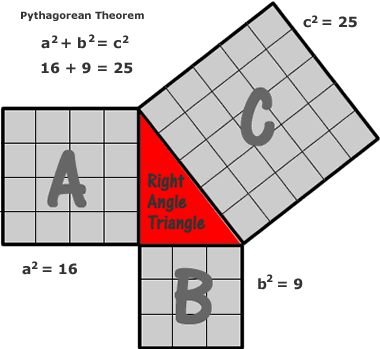4.2.Proof:(Euclid's proof)
a) We label a few points (see the diagram), and draw lines BI and CE. ΔACE is congruent to ΔAIB, by the SAS theorem, because AC=AI, AE=AB and CAE=IAB (both angles are congruent to angle CAB + a right angle).
b) But, the area of ΔAIB is equal to half the area of square ACHI (They have the same base AI and height IH).
c) The area of triangle ACE is equal to half the area of the rectangle AGFE. So, the square ACHI is equal, in area, to the rectangle AGFE.
d) Likewise, we show that the area of the smaller square is equal to the area of the smaller rectangle.So, the area of the large square is equal to the sums of the areas of the two smaller squares.
e) Hence proved the pythagoras theorem.

4.3 Converse of pythagoras theorem: If in a triangle, square of one side is equal to the sum of the squares of the other two sides, then the angle opposite the first side is a right angle.

4.4 Problems:

Q1. Jake leaves school to go home. He walks 6 blocks North and then 8 blocks west. How far is Jake from the school?

Q2. Find the value of x and y in the figure below.

Q3.Find the value of AC, Given that C is the midpoint of BD.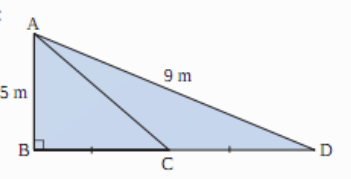Q4. Which of the following triangles a,b,c is a right angled triangles?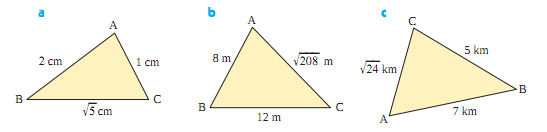Q5. In the figure below show that Area A + Area B = Area C.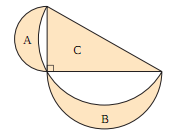Q6. An aircraft hangar is semi-cylindrical with diameter 40 m and length 50 m. A helicopter places a cable across the top of the hangar, and
one end is pinned to the corner at A. The cable is then pulled tight and pinned at the opposite corner B. Determine the length of the cable.

### Angle: The angle formed by the two adjacent sides of the polygon is called the angle of the polygon.

5.2.Kinds of Polygon:

a)Convex:If each interior angle of a polygon is less than 180°, the polygon is convex. If for each side of the polygon, the line containing that side has all the other vertices on the same side of it.

b)Concave:  If at least one interior angle of a polygon is greater than 180°,. the polygon is concave.

c)Regular: If all the sides of a polygon are equal and all the angles are equal, the polygon is called a regular polygon.

5.3 Angle Property:
a)Interior angle of a regular polygon with n sides is (n-2)*180/n.
b)Exterior angle is 360 - interior angle.
c) In a convex polygon of n side, the sum of interior angles is equal to (2n - 4) right angles.
d) The sum of exterior angles of a polygon is equal to 4 right angles.

5.4 The figures of values regular polygon are shown from n=3 to n=10.

5.5 Condition for a quadrilateral to be:
a)Parallelogram:
i)Its opposite sides are equal. or,
ii)Its opposite angles are equal. or,
iii)its diagonal bisects each other. or,
iv)It has one pair of opposite sides equal and parallel.

b)Rhombus: If in a parallelogram, the diagonals bisect each other at 90°, then its a rhombus.

c)Rectangle: If in a parallelogram the diagonals are equal then its a rectangle.Here PR=QS.

d)Square: The diagonals are equal and bisect each other at right angles.

5.5.Construction of Quadrilateral:To construct a quadrilateral five elements show be know.They can be from one of these sets:
a)Four sides and a diagonal.
b)Three sides and both diagonals.
c)Two adjacent sides and three angles.
d)Three sides and two included angles.
e)Four sides and an angle.

Construction of a parallelogram with a two given sides and an angle:
a)Draw the base AB with given length.
b)Make the angle A.
c)Cut off the arc of length along the ray AD.
d)From B and D cut off arcs of the respective lengths to find the other vertex of the parallelogram.

5.6.Area theorems on parallelogram:
a)Parallelogram on the same base and between the same parallels are equal in area.
b)The area of a triangle is half that of a parallelogram on the same base and between that same parallels.Area(ΔEAB) = ½ area(ABCD)

c)Triangles between the same base and between the same parallels are equal in area. ΔABD and ΔABC have the same base and are between the same parallels, thus area(ΔABD) = (ΔABC).
d)Triangles with equal areas on the same base have equal corresponding altitudes. In the figure below ΔQPQ and ΔBPQ are equal in area with the common base PQ.

5.7. Problems:

Q1. ABCD is a parallelogram. What special name will you give it if the following facts are known?(i) AB = AD (ii)∠DAB = 90° (iii) AB = AD and DAB = 90°.

Q2.In the figure PQRS is a parallelogram and X, Y are the points on the diagonal QS, such that SX = QY. Prove that quadrilateral PYRX is a parallelogram.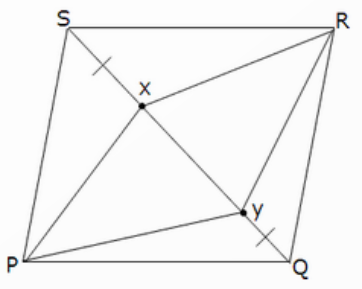Q3.ABCD is a parallelogram. E and F are the midpoints of the sides AB and CD respectively. Prove that the segments CE and AF trisect the diagonal BD.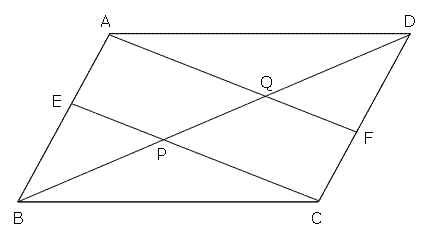Q4.Suppose that the lengths of two diagonals of a parallelogram are 8 cm and 6 cm and the angle between them is 60°.Construct the parallelogram.

Q5.ABCD is a parallelogram and line segments AX and CY bisect A and C respectively. Prove that AX || CY.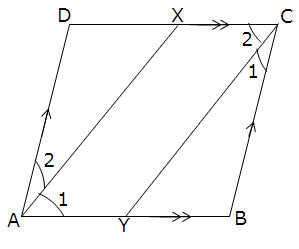Q6.In the figure below, PQRS and ABRS are parallelograms and X is any point on side BR. Show that area(ΔPXS)= ½ area(PQRS)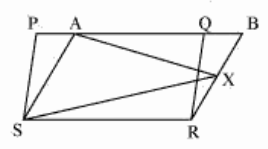### 6.Construction

6.1.Angles:
1)Constructing 60°:
Step 1:  Draw the arm PQ.
Step 2:  Place the point of the compass at P and draw an arc that passes through Q.
Step 3:  Place the point of the compass at Q and draw an arc that passes through P.  Let this arc cut the arc drawn in Step 2 at R.
Step 4:Join P and R and angle QPR=60°.
It is as shown below:

2)Constructing 30°:
We know 30° = ½ 60°
So, to construct an angle of 30º, first construct a 60º angle and then bisect it. To bisect an angle we follow the rules below:
Step 1:  Draw the arm PQ.
Step 2:  Place the point of the compass at P and draw an arc that passes through Q.
Step 3:  Place the point of the compass at Q and draw an arc that cuts the arc drawn in Step 2 at R.
Step 4:  With the point of the compass still at Q, draw an arc near T as shown.
Step 5:  With the point of the compass at R, draw an arc to cut the arc drawn in Step 4 at T.
Step 6:  Join T to P.  The angle QPT is 30º.
It is shown below

3)Constructing 120º:
a) We know that 120º is the supplement of 60º, so make 120º, make 60º and extend its arm.

4)Constructing 90º:
Step 1:  Draw the arm PA.
Step 2:  Place the point of the compass at P and draw an arc that cuts the arm at Q.
Step 3:  Place the point of the compass at Q and draw an arc of radius PQ that cuts the arc drawn in Step 2 at R.
Step 4:  With the point of the compass at R, draw an arc of radius PQ to cut the arc drawn in Step 2 at S.
Step 5:  With the point of the compass still at R, draw another arc of radius PQ near T as shown.
Step 6:  With the point of the compass at S, draw an arc of radius PQ to cut the arc drawn in step 5 at T.
Step 7:  Join T to P. The angle APT is 90º.

5)Constructing 45º:
We know 45º = ½ 90º. So we just bisect 90º using the steps used before.

6)Constructing 135º:
We know that 135º is 90º + 45º. Thus we can draw 90º from the above methods and then 45º on it to make it 135º, as shown in the figure.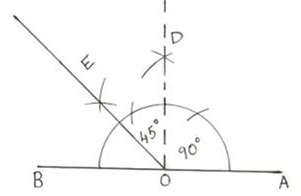7)Constructing 75º:
Make 90º and from that subtract 15 degree, which can be constructed by bisecting 30º and then further bisecting it to 15º, as shown in the figure.6.2.Construct tangents to a circle from an external point:
Step 1: Draw a straight line between the center O of the given circle and the given point P.
Step 2: Find the midpoint of this line by constructing the line's perpendicular bisector. The midpoint may be inside or outside the circle, depending on the circle size and the location of the given point.
Step 3: Place the compass on the midpoint just constructed, and set it's width to the center O of the circle.
Step 4:  Without changing the width, draw an arc across the circle in the two possible places. These are the contact points J, K for the tangents.
Step 5:  Draw the two tangent lines from P through J and K.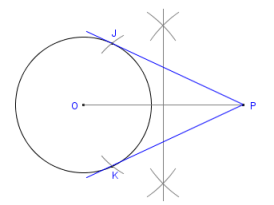6.3.Circumcircle of a triangle:
Step 1: Find the bisector of one of the triangle sides. Any one will do. See Constructing the Perpendicular Bisector of a Line Segment.
Step 2:  Repeat for the other side. Any one will do.
Step 3: The point where these two perpendiculars intersect is the triangle's circumcenter, the center of the circle we desire.
Step 4:  Place the compass point on the intersection of the perpendiculars and set the compass width to one of the points A,B or C. Draw a circle that will pass through all three.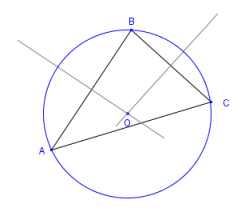6.4.Incircle of a triangle:
Step 1: Place the compass point on any of the triangle's vertices. Adjust the compass to a medium width setting. The exact width is not important.
Step 2:  Without changing the compass width, strike an arc across each adjacent side.
Step 3:  Change the compass width if desired, then from the point where each arc crosses the side, draw two arcs inside the triangle so that they cross each other, using the same compass width for each.
Step 4: Using the straightedge, draw a line from the vertex of the triangle to where the last two arcs cross.
Step 5:  Repeat all of the above at any other vertex of the triangle. You will now have two new lines drawn.
Step 6: Where the two new lines intersect, mark a point as the incenter of the triangle.
Step 7:  Draw the perpendicular from the incenter to a side of the triangle. Label the point where it meets the side M.
Step 8:  Place the compass on the incenter and set the width to point M. This is the radius of the incircle, sometimes called the inradius of the triangle.
Step 9: Draw a full circle, to obtain the in-circle.

6.4.Constructing a regular Hexagon:
Step 1: We start with a line segment AF. This will become one side of the hexagon. Because we are constructing a regular hexagon, the other five sides will have this length also.
Step 2: Set the compass point on A, and set its width to F. The compass must remain at this width for the remainder of the construction.
Step 3 :  From points A and F, draw two arcs so that they intersect. Mark this as point O.This is the center of the hexagon's circumcircle.
Step 4: Move the compass on to A and draw an arc across the circle. This is the next vertex of the hexagon.
Step 5:  Continue in this way until you have all six vertices. (Four new ones plus the points A and F you started with.)
Step 6: Draw a line between each successive pairs of vertices. And the figure is formed.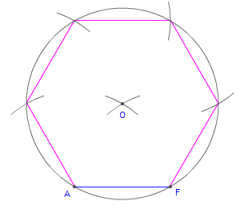6.5.Problems:

Q1.Construct the following angles:
a)225°
b)150°
c)210°
d)225°
e)245°
Q2.Use a compass and a ruler to construct a triangle ABC with AB=8 cm, BC= 6 cm and ∠ABC=90°.Measure ∠BCA and ∠ACB.

Q3.Construct a trapezium DEFG with DE=6.5 cm, ∠DEF=90° EF=5.5 cm, ∠EFG=90° and ∠EDG=60°.

Q4.Construct a Hexagon to of side 6.5 cm.

Q5.Construct the incircle and the circumcircle of a triangles whose one side is 5 cm , another side is 6 cm and the angle between them is 120°.

## 7.Locus

7.1.Definition: A locus is set of points that share a property.For example a circle may be defined as a loci of all the points in a plane at a fixed distance from a point(center).

7.2Theorems:
a)Theorem 1:The locus of points at a fixed distance, d, from point P is a circle with the given point P as its center and d as its radius. The loci is shown in the figure below.

b)Theorem 2:The locus of points equidistant from two intersecting lines, l1 and l2, is a pair of bisectors that bisect the angles formed by l1and l2 .

c)Theorem 3:The locus of points equidistant from two points, P and Q, is the perpendicular bisector of the line segment determined by the two points.

7.3.Problems:
Q1. Given 2 points A and B, what is the locus of points P so that angle APB is a right angle?

Q2. A 10-foot ladder is placed vertically against a wall, and then the foot of the ladder is moved outward until the ladder lies flat on the floor with one end touching the wall.  What is the locus of the midpoint of the ladder as it slides?

Q3.If A and B are two points that are 2 units apart, what is the locus of points that are 1 unit from A and 2 units from B?

Q4.What is the locus of the centers of circles that are tangent to a given line at a given point?

Q5. What is the locus of the extremities of line segments meeting at a given point and bisected by a line not through the given point?

Q6.Given AB perpendicular to BC. D is on AB and E id on BC. DE is of a given length.
i)What is the locus of the midpoint of DE?
ii)What is the locus of a point on DE one-fourth the distance from D to E?
iii)What is the locus of a point on DE three-fourths the distance from D to E?
iv)What is the locus of a point on DE that is five-fourths the distance from E to D?

## 8.Circle: Chord and Chord Properties

8.1.Definitions:
a)Circle: It is a simple shape of Euclidean geometry consisting of those points in a plane that are equidistant from a given point, the centre. The distance between any of the points and the centre is called the radius.

b)Chord: A chord of a circle is a geometric line segment whose endpoints both lie on the circumference of the circle.

The figure below shows the required parameters.

8.2.Chord Properties:
a)A straight line from the center of the circle to a chord, which bisects the chord which is not a diameter, is perpendicular to the chord. This can be proved by SSS congruence of triangles.In the figure AP=BP, thus OPA=90°.

b) A perpendicular from the center to a chord bisects the chord.This is the converse of the previous property. Here OC is perpendicular to AB at C and AC=CB, which follows from the property.

c) Equal Chords are equidistant from the center.In the figure below AE=BF, and from the property OC=OD.This can be proved using RHS congruacy in ΔOAC and ΔOBD.

d)Chords which are equidistant from the center are equal.In the figure below AF=AE, thus from the property BG=DC.

e)There is one and only one circle passing through given three points.In the figure below the circle shown is the only circle that can pass through the points 1,2 and 3.

8.3.Problems:
Q1. Radius CD bisects chord AB. Chord AB measures 8 cm. The radius of the circle is 5 cm. What is the length of line segment CE? Justify your solution.

Q2) Find the value of x in the following figures:

Q3) Line segment OC is a bisector of chords AB and DE. If O is the centre of the circle on the left and C is the centre of the circle on
the right, explain how you know that AB is parallel to DE.

Q4)The circular cross section of a water pipe contains some water in the bottom. The horizontal distance across the surface of the
water is 34 cm. The inner diameter of the pipe is 50 cm. What is the maximum depth of the water?

Q5) In this circle, the diameter AE = 20 cm, the chord DE = 16 cm, AF = 5 cm, and ∠BFE = 90°.Determine the following measures and

Q6)Some plastic tubing is moulded with an I-beam on the inside to provide extra strength. The length of each of two parallel
chords is 10 mm, and the perpendicular distance between these two chords is 12 mm. What is the diameter of the circular tubing?

## 9.Circle: Arc and Chord Properties

9.1.Definitions:
a)Arc: An arc is a portion of the circumference of a circle.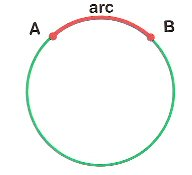9.2.Properties:
a)The angle that an arc at the center subtends is double that which it subtends at any point on the circumference.This can be seen in the figure below.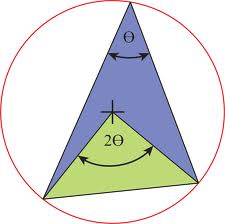b)Angles in the same segment are equal.In the figure below, on the segment AB, ∠ADB = ∠ACB.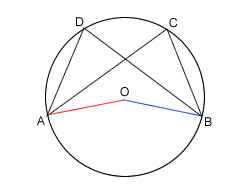c)Angle in a semicircle is a right angle. In the figure below the angle ∠APB = 90°.d)If two arcs subtend equal angles at the center, then they are equal.In the figure below ∠AOB=∠DOC (angles at the center), then from the property the arcs arc(AB)=arc(DC).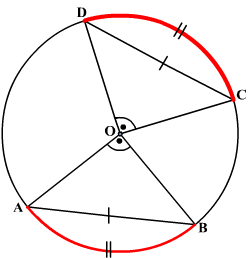e) If two arcs are equal then they subtend equal angles at the center. It is the converse of the above property, which can also be seen from the above figure.

f) If two Chords are equal, then they cut off equal arcs. And its converse also holds. In the figure below, arc(AB)=arc(DC), so from property AB = DC.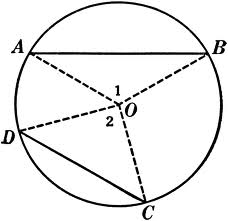g) If two chords intersect internally or externally then the product of the lengths of the segments are equal.  In the figure shown below, the chords AB and CD intersect at P, so PA.AB = PC.CD which follows from the property.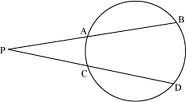9.3.Problems:
Q1)In the figure below, arc AB = 60° and arc CD = 100°.Find the value of the angle marked as 1.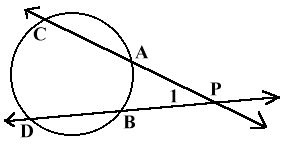Q2)In Figure shown below, O is the centre of the circle and∠AOC = 120°. Find ∠ABC.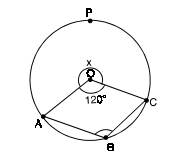Q3)In Figure shown below, O is the centre of the circle and AD bisects ∠BAC. Find ∠BCD.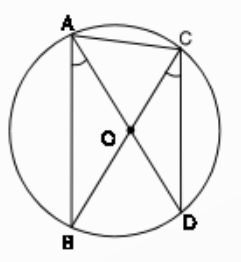Q4)In Figure shown below, O is the centre of the circle, ∠POQ = 70° and  PS OQ ⊥ . Find ∠MQS.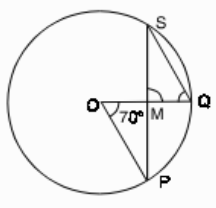Q5)Two circle intersect in A and B. AC and AD are the diameters of the circles. Prove that C, B and D are collinear.See the figure below.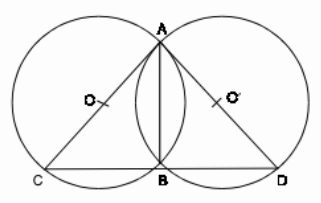Q6)In Figure 19.30, C1 and C2 are two circles with centre O1  and O2 and intersect each other at points A and B. If O1O2  intersect AB at M then show that:
(i) ∆O1AO2  ≅ ∆O1BO2
(ii) M is the midpoint of AB
(iii) AB O O ⊥ 1 2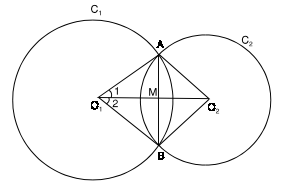### 10.Circle: Cyclic and Tangent Properties

10.2.Definitions:
a)Cyclic Quadrilateral: If there exists a circle that can be drawn, such that all the point of the quadrilateral lie on the circle then the quadrilateral is a cyclic quadrilateral.In the figure show, ABCD is a cyclic quadrilateral.\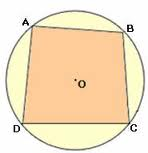b)Tangent:A line, line segment, or ray that touches a circle at exactly one point.In the figure below, the ray cuts the circle at only one point called the point of tangency.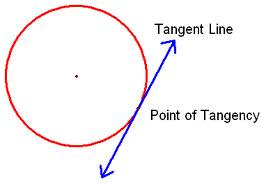10.2.Cyclic Properties:
a)Opposite angles of a cyclic quadrilateral are supplementary. In the figure shown below , ABCD is a cyclic quadrilateral, and its opposite angles are supplementary. ∠ADC + ∠ABC = 180, which can be seen in the figure.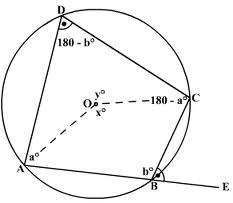b)The exterior angle of a cyclic quadrilateral is equal to the opposite interior angle.In the figure below, ∠CBA = ∠ADE, from the property mentioned.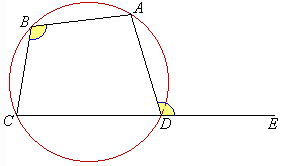10.2.Tangent Properties:
a)The tangent at any point of a circle and the radius throught that point make an angle of 90°. In the figure below,  AC ⊥ BO, AC is the tangent and OB is the radius.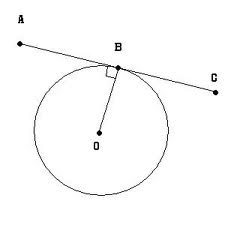b)If two circles touch each other, then the point of contact lies on the line which passes through the centers of the two circles. In the figures below, the line joining the centers passes through the point of contact.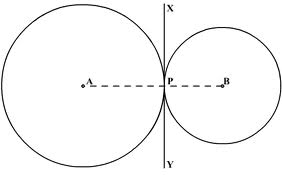c)From any point outside the circle two tangents can be drawn to a circle, which are equal in length.It can be proved by RHS condtion as shown in the figure below.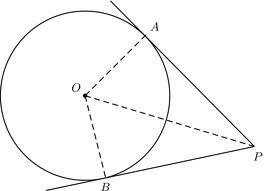d)If a chord and a tangent intersect externally, then the product of the length of segments of the chord is equal to the square of the length of the tangent from the point of contact to the point of intersection. In the figure below, OA.OB = OC².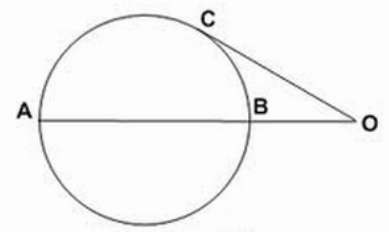e)If a line touches a circle and from that point a chord is drawn, the angles between the tangent, and the chord are respectively equal to the angles, in the corresponding alternative segments. In the figure below it can be seen that angle in the segment is equal to the angle between the chord and the tangent.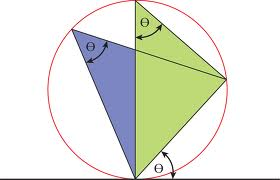10.3.Problems:
Q1)In Figure shown below, PQRS is a cyclic quadrilateral whose diagonals intersect at A. If ∠SQR = 80° and ∠QPR = 30°, find ∠SRQ.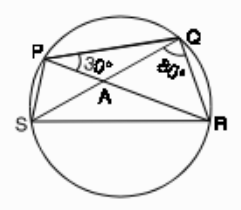Q2)In Figure shown below, PQRS is a cyclic quadrilateral, and the side PS is extended to the point A. If ∠PQR = 80°, find ∠ASR.

Q3)In the figure below, triangle ABC is tangent to the circle of center O at two points. The lengths of AM and BC are equal to 6 and 18 cm respectively. Find the radius of the circle.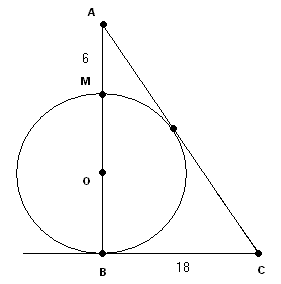Q4) What is the perimeter of the triangle in the figure shown below.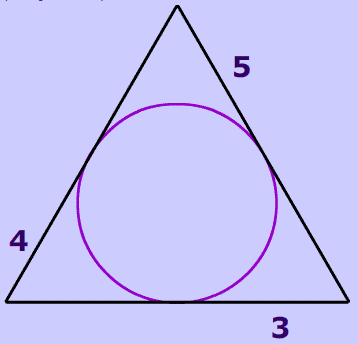Q5)The figure shown, circles C1 and C2, tangent at C. Diameter DE extended and FG are perpendicular. If FG is tangent to C2, at G. Prove that the points D, C, and G are collinear.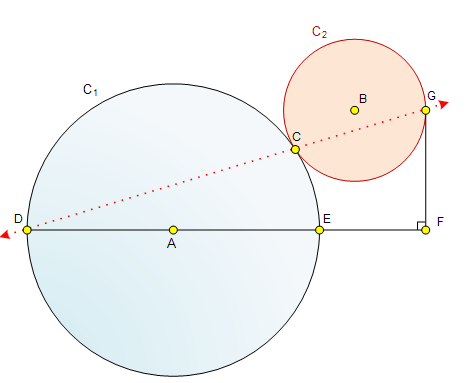Q6)In the figure shown below, AB is tangent to the circle at point B. BD is a diameter of the circle. AB = 7 mm and AD = 25mm, and BCE is an equilateral triangle.
a) What is the length of diameter BD?
b) What is the length of the chord BE?
c) What is the measure of the inscribed angle ∠BED?
d) What is the length of chord DE?